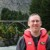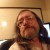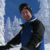# Sigma Performance Levels

Six Sigma – iSixSigma Forums General Forums New to Lean Six Sigma Sigma Performance Levels

Viewing 4 posts - 1 through 4 (of 4 total)
• Author
Posts
• #56077Shareef Zighary
Guest

Hello Everyone,

I’m relatively new to the world of Six Sigma and I’m struggling to understand whether the empirical rule is related to Sigma performance levels or not.

I understand how to calculate the Baseline Sigma through techniques such as DPMO and how this translates to a specific Sigma Performance Level, but I was recently introduced to another technique for calculating Sigma Performance and this is the Z score.

So, after diving into this technique I understood that a Z score just denotes Sigma Performance i.e. the higher the Z score then the better the process is performing. I’m also aware of short-term vs long term and Sigma Shift. What confuses me however is that if I calculate a Z score of 2, does this mean that I can expect a yield of 69% and a defect rate of 31% because my process is operating at a performance level of 2 Sigma? Or, does this mean that I can expect a yield of 95% based on the empirical rule that 95% of the data falls between +/- 2 standard deviations from the mean? I’m sure it’s the former, but I guess I’m confused as to whether the empirical rule is related to Sigma Performance.

As you can see this question may be confusing because I’m relatively new, but I would really appreciate the support to understand whether I really need to consider the empirical rule when calculating a Sigma Performance level, or just rely on the DPMO conversion to Sigma Performance.

Thanks!

0
#202981Chris Seider
Participant

The Z score tells you what dpmo is in the defective portion of the normal distribution or ppm defective depending on your data.

The sigma lines on an SPC chart are RELATED but based on differently ran statistics such as Xbar or Rbar or Sbar or …

0
#202982Strayer
Participant

Sigma level is the probability that a single measurement will be within specification limits, expressed as the number of standard deviations between the mean and the nearest spec limit. Z-score is the probability of where any single measurement will fall within the distribution of the sample, expressed as the number of standard deviations from the mean, without regard to spec limits.

0
#202985Chuck White
Participant

Strayer is correct — Z-score is just a unit conversion, often used in interim steps of capability calculations, but not a measure of capability in itself. However, I think you might be confusing the terms Z-score and Z-bench. Z-bench is the calculation of the Sigma level.

Getting to the root of your question, the main difference between the 69% and the 95% that you referenced is the 1.5 Sigma Sift that is typically assumed. Sigma Shift represents the additional long term variation that most processes have.

In Six Sigma, we typically express capability in terms of short term Z-bench (Sigma level), and long term DPMO. So if your capability study was based on short term data, a short term Z-Bench of 2 would correspond to a long term DPMO of 308,500. However, if your data was long term, your short term Z-Bench would be 3.5 (2 + 1.5 Sigma Shift), and your long term DPMO would be 22,750 (closer to 2.5% than 5% since Z-Bench takes both sides of the distribution into account).

0
Viewing 4 posts - 1 through 4 (of 4 total)

You must be logged in to reply to this topic.